The lengths of the sides of a rectangle are a and b, where a > b The sum of the lengths of the two shorter sides and one of the longer sides of the rectangle is 36. What value of a maximizes the area of the rectangle?

A .9
B. 12
C. 18
D. 24

The answer is C. I suspect there is an easier way to solve than completing the square and finding the vertex of the resulting quadratic function. What is your most direct, easy to understand solution to this calculator-allowed question?

This is an interesting one. The wording is a little off because there are non-answer choices that would better “maximize” the area of the rectangle, but more on that at the end. Question construction quibbles aside, my first instinct is to backsolve.

Translating “the sum of the lengths of the two shorter sides and one of the longer sides of the rectangle is 36,” we get. The answer choices give us values for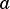, so we can figure out the corresponding value offor each choice:

A)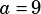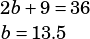This doesn’t work because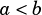in this choice, while the question tells us.

B)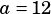This doesn’t work because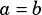in this choice, while as noted above, the question tells us.

C)This gives us a rectangle area of.

D)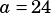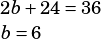This gives us a rectangle area of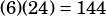.

Because 162 > 144, C is the best answer.

Now, back to “maximizing.” It’s a useful bit of trivia to know that, given a set perimeter, (like if you had a certain number of fence lengths to work with to build a rectangular fence) the maximum rectangular area will be when you have a square. The further apart the length and width are, the lower the area will be.

In this question, because we’re told that, we know we’re dealing with an amount of length and width that can’t change—asgets bigger,gets smaller, and vice versa. So we know without checking all choices that the greatest area will be when the length and width are closest (without breaking therule).

The reason I don’t like the author’s choice of the word maximizing here is that choice B doesn’t work because, but, or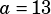if we’re sticking to integers, would do better to maximize the area. The question should really have just asked which choice produces the greatest rectangle area.Robin says: